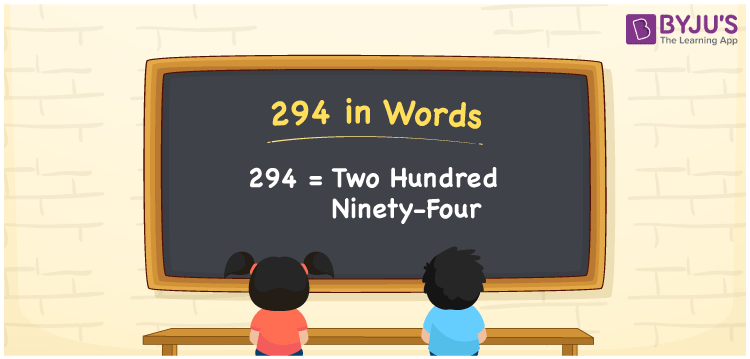# 294 in Words

294 in words is written as Two hundred ninety-four. In both the International System of Numerals and the Indian System of Numerals, 294 is written as Two hundred ninety-four. The number 294 is a Cardinal Number as it represents some quantity. For example, the “cost of the dress is 294 rupees”.

 294 in Words Two hundred ninety-four Two hundred ninety-four in Number 294

## 294 in English Words

We write 294 in Words using the letters of the English alphabet. Therefore, we read 294 in English as “Two hundred ninety-four”.## How to Write 294 in Words?

To write 294 in words, we shall use the place value chart. In the place value chart, write 2 in the hundreds, 9 in the tens, and 4 in the ones, respectively. Now let us make a place value chart to write the number 294 in words.

 Hundreds Tens Ones 2 9 4

Thus, we can write the expanded form as

2 × Hundred + 9 × Ten + 4 × One

= 2 × 100 + 9 × 10 + 4 × 1

= 200 + 90 + 4

= 294

= Two hundred ninety-four.

294 is a natural number, the successor of 293 and the predecessor of 295.

294 in words – Two hundred ninety-four

• Is 294 an odd number? – No
• Is 294 an even number? – Yes
• Is 294 a perfect square number? – No
• Is 294 a perfect cube number? – No
• Is 294 a prime number? – No
• Is 294 a composite number? – Yes

## Frequently Asked Questions on 294 in Words

Q1

### How to write 294 in words?

294 in words is written as Two hundred ninety-four.
Q2

### How to write 294 in the International and Indian System of Numerals?

In both, the system of numerals, 294 in words, is written as Two hundred ninety-four.
Q3

### What is the successor of 294?

The successor of 294 is 295.